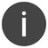# CVTIZV

Pass an integer value to a zoned numeric value

 WTSupported in traditional Synergy on Windows USupported on UNIX VSupported on OpenVMSThe CVTIZV routine was deprecated in Synergy/DE version 9; we recommend you use an integer assignment instead, as shown in the note in the Discussion.
```xcall CVTIZV(value, field)
```

Arguments

value

Passed directly or using one of the following functions: (n)

^VAL = Pass the integer by value.

^REF = Pass the integer by reference.

^XTRNL = Pass the integer by external reference. (OpenVMS only)

field

The variable that will be loaded with the converted integer. (n)

DiscussionWe recommend that you replace the deprecated CVTIZV routine with an integer assignment. For example, xcall cvtizv(^ref(prcnmlen),retlen) would become ```retlen = ^addr(prcnmlen) ``` xcall cvtizv(^val(numeric field), retlen) would become ```retlen = numeric field ``` xcall cvtizv(^xtrnl(SOME LITERAL), retlen) would become ```retlen = ^xtrnl(SOME LITERAL) ``` See Moving integer data to a decimal/packed destination for more information about assigning integer values.

The CVTIZV subroutine converts an integer value to a zoned numeric value. The integer must be passed by value, reference, or external reference.

A field can be converted either into itself or into another named field.

Examples

The following example converts the externally defined constant SS\$_ENDOFFILE and places the result in the variable sseof. SS\$_ENDOFFILE is a system service condition value.

```xcall cvtizv(^xtrnl(SS\$_ENDOFFILE), sseof)
```